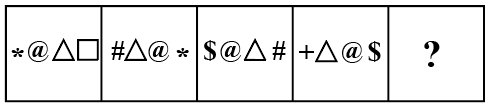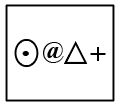Question 2

# Select the figure that will come next in the following figure series.Solution

Given Figure,In the given figure follow a pattern then next  is follow pattern in the given optiontherefore? = D Ans

• Free SSC Study Material - 18000 Questions
• 230+ SSC previous papers with solutions PDF